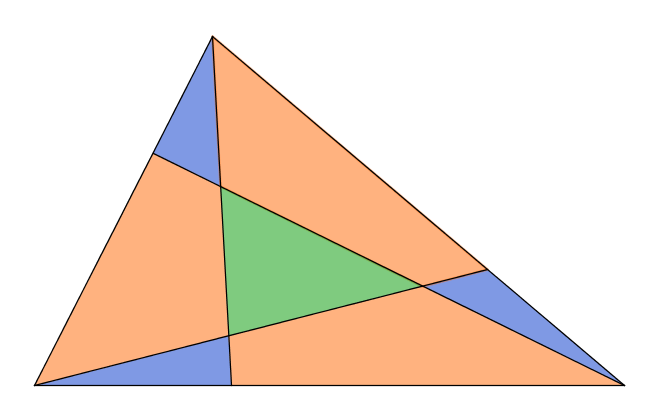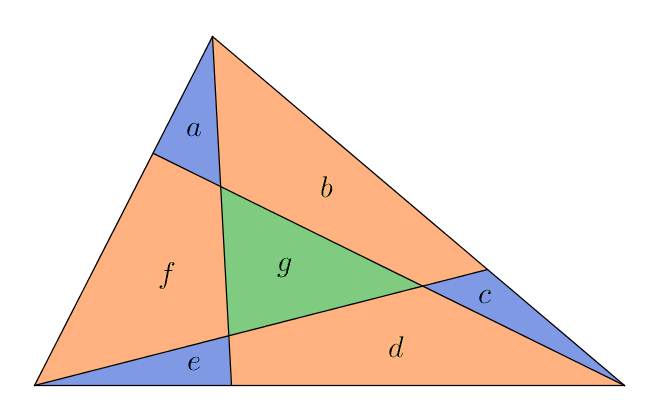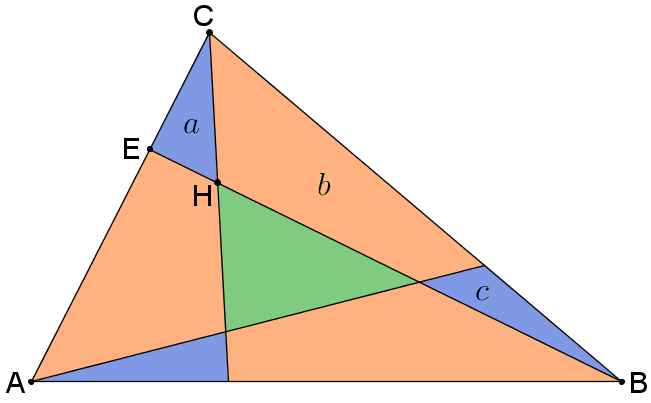You may also likeAreas and Ratios

Do you have enough information to work out the area of the shaded quadrilateral?Napoleon's Hat

Three equilateral triangles ABC, AYX and XZB are drawn with the point X a moveable point on AB. The points P, Q and R are the centres of the three triangles. What can you say about triangle PQR?Plane to See

P is the midpoint of an edge of a cube and Q divides another edge in the ratio 1 to 4. Find the ratio of the volumes of the two pieces of the cube cut by a plane through PQ and a vertex.

Another Triangle in a Triangle

Age 16 to 18Challenge Level
You may wish to look at Triangle in a Triangle and Areas and Ratios before exploring this problem.

In the diagram below, points have been chosen that are one third of the way along each side. Each of these points is then joined to the opposite vertex.What fraction of the area of the whole triangle is the area of the green triangle?

If you are not sure how to get started, click below to read some thoughts, hints and suggestions that might be helpful. Before moving onto the next hint, do as much as you can with the information you have already worked out.

The base of triangle $a+f+e$ is $\tfrac{1}{3}$ of the base of the whole triangle.

Can you use this to work out the area of $a+f+e$ as a fraction of the whole triangle?What about $a+b+c$ and $c+d+e$?

We have three triangles, each with an area of $\tfrac{1}{3}$ of the whole triangle.

But none of these tell us anything about $g$.

But we do know that $a$, $b$, $c$, $d$, $e$, $f$ and $g$ together make the whole triangle!

Can we combine all this to find an expression for $g$ in terms of other areas?

It would be useful to know the areas of the little blue triangles...We know that:
• $a$ has the same height as triangle $ECB$.
• Triangle $ECB$ is $\tfrac{1}{3}$ of the whole triangle

If we can find the length $EH$ as a fraction of $HB$, we could work out the area of the blue triangle...

Sometimes it helps to add in an extra line...Can you find some similar triangles in this diagram?

How does $EJ$ compare with $AF$?

How does $EJ$ compare with $BF$

How does $EH$ compare with $HB$?

How does $EH$ compare with $EB$?

An alternative method for solving this problem is to use vectors.